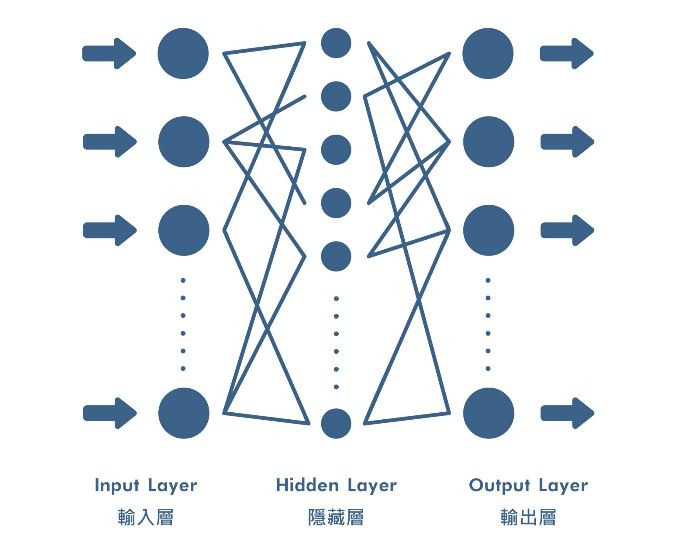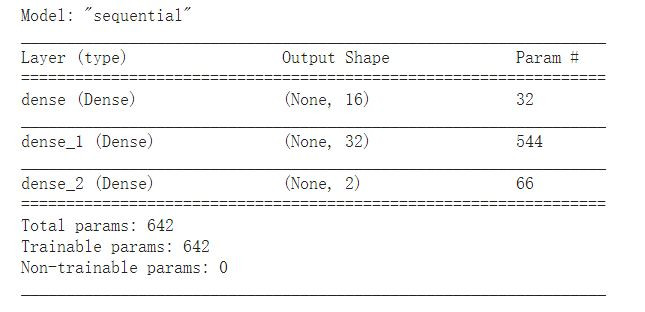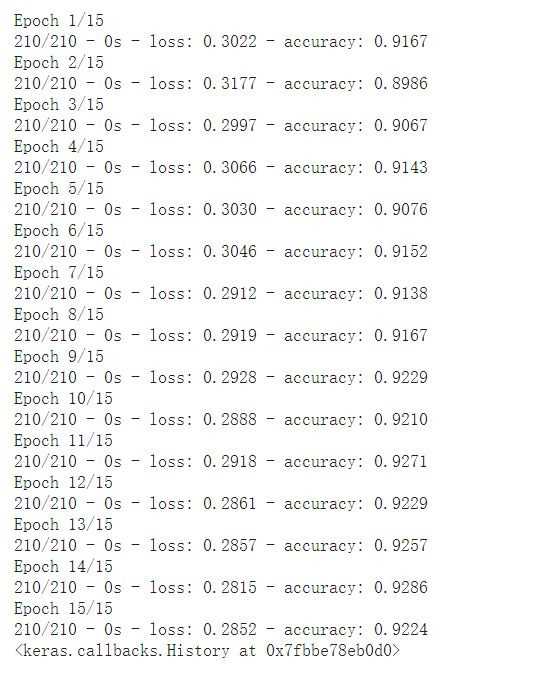#DAY 20
0
AI & Data

## 一、神經網路組成## 二、使用動手架一個神經網路

### 首先載入一些基本套件

``````import tensorflow as tf
from tensorflow import keras
from tensorflow.keras.models import Sequential
from tensorflow.keras.layers import Activation, Dense

from tensorflow.keras.metrics import categorical_crossentropy
``````

### 架設一個神經網路模型

``````model = Sequential([
Dense(units=16, input_shape=(1,), activation='relu'),
Dense(units=32, activation='relu'),
Dense(units=2, activation='softmax')
])
``````

### 查看模型架構

``````model.summary()
#param代表總參數量
``````### 編譯模型

``````model.compile(
loss='sparse_categorical_crossentropy',
metrics=['accuracy'])
``````

optimizer：一種優化器，主要幫助是模型梯度下降時能找到最優解。

learning_rate：學習率，模型收斂的速度

loss：損失函數，模型的損失值越小越好

metrics：評估指標，使用Accuracy來評估

## 三、訓練模型

### 創建資料集

``````import numpy as np
from random import randint

train_samples = []
train_labels = []

for i in range(50):
# 5%的年輕人會有不適症狀
random_young = randint(1,30)
train_samples.append(random_young)
train_labels.append(1)

# 5%的年長者不會有不適症狀
random_old = randint(31,100)
train_samples.append(random_old)
train_labels.append(0)

for i in range(1000):
# 95%的年輕人不會有不適症狀
random_young = randint(1,30)
train_samples.append(random_young)
train_labels.append(0)

# 95%的年長者會有不適症狀
random_old = randint(31,100)
train_samples.append(random_old)
train_labels.append(1)
``````

### 打亂資料

``````from sklearn.utils import shuffle
train_samples = np.array(train_samples)
train_labels = np.array(train_labels)
train_samples, train_labels  = shuffle(train_samples, train_labels)
``````

### 模型訓練

``````model.fit(train_samples,train_labels,batch_size=10, epochs=5, verbose=2)
``````